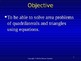# 6th Grade Geometry 4 - Using Equations to Solve Area Problems Powerpoint LessonSubject
File Type

Presentation (Powerpoint) File

(4 MB|45 pages)
Standards
Also included in:
1. Common Core Sixth Grade Geometry Bundle is made up of 8 different lessons comprising 407 slides. These lessons cover all of the Common Core Standards in 6th Grade Geometry. The lessons included are Geometry 1 - Area of Triangles, Geometry 2 - Area of Rectangles and Squares, Geometry 3 - Area of Pa
\$28.00
\$22.40
Save \$5.60
2. Sixth Grade Powerpoint Math Bundle is composed of 58 different high quality math powerpoint lessons comprising 2,923 slides. There are 13 strategies of effective teaching based on research in these powerpoint lessons. . For further information on each lesson click on them individually. This is a
\$203.00
\$142.10
Save \$60.90
• Product Description
• Standards

Sixth Grade Geometry 4 - Using Equations to Solve Area Problems teaches students how to find the missing information in area problems of squares, parallelograms, trapezoids and triangles using equations. There is also a review of the following area formulas: rectangles, squares, parallelograms, rhombuses, trapezoids and triangles. There are 13 strategies of effective teaching based on research. For best results, students should have white boards and dry erase markers. Included in this powerpoint lesson are the following:

• title slide
• focus slide
• objective
• Students will be able to solve area problems of quadrilaterals and triangles using equations.
• essential question
• How can I solve area problems of quadrilaterals and triangles using equations?
• vocabulary
• concept development
• step-by-step demonstrations on how to solve area problems of quadrilaterals and triangles using equations
• guided practice
• checking for understanding
• problem solving with word problem
• reward slide

Don't forget to leave feedback on this lesson to earn points for purchasing other TpT products.

You may be interested in the following related powerpoint lessons:

Link - Common Core 6th Geometry 1 - Area of Triangles

Link - Common Core 6th Geometry 2 - Areas of Rectangles and Squares

Link - Common Core 6th Geometry 3 - Areas of Parallelograms, Rhombuses and Trapezoids

Link - Common Core 6th Geometry 5 - Volume

Link - Common Core 6th Geometry 6 - Polygons in the Coordinate Plane

Link - Common Core 6th Geometry 7 - 3D Figures (Solids) and Their Nets

Link - Common Core 6th Geometry 8 - Surface Area Using Nets

Solve real-world and mathematical problems by writing and solving equations of the form 𝘹 + 𝘱 = 𝘲 and 𝘱𝘹 = 𝘲 for cases in which 𝘱, 𝘲 and 𝘹 are all nonnegative rational numbers.
Use variables to represent numbers and write expressions when solving a real-world or mathematical problem; understand that a variable can represent an unknown number, or, depending on the purpose at hand, any number in a specified set.
Find the area of right triangles, other triangles, special quadrilaterals, and polygons by composing into rectangles or decomposing into triangles and other shapes; apply these techniques in the context of solving real-world and mathematical problems.
Total Pages
45 pages
N/A
Teaching Duration
55 minutes
Report this Resource to TpT
Reported resources will be reviewed by our team. Report this resource to let us know if this resource violates TpT’s content guidelines.